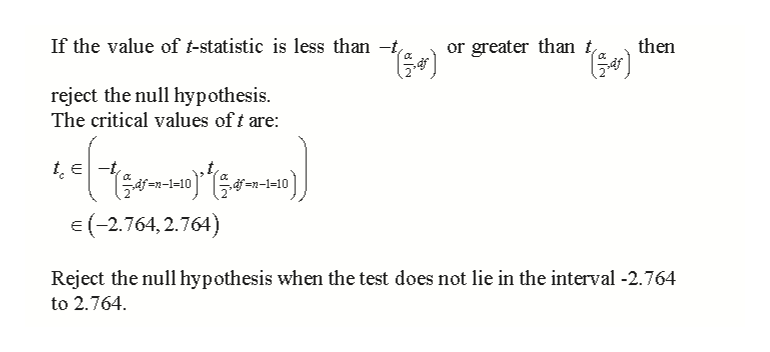# Given the following hypotheses: H0: μ = 550H1: μ ≠ 550A random sample of 11 observations is selected from a normal population. The sample mean was 556 and the sample standard deviation 7. Using the 0.01 significance level:a) State the decision rule. (Negative amount should be indicated by a minus sign. Round your answers to 3 decimal places.)Reject H0 when the test statistic is _____________ the interval (_____, _____)b) Compute the value of the test statistic. (Round your answer to 3 decimal places.)Value of the test statistic _______________

Question
4 views

Given the following hypotheses:

H0: μ = 550

H1: μ ≠ 550

A random sample of 11 observations is selected from a normal population. The sample mean was 556 and the sample standard deviation 7. Using the 0.01 significance level:

a) State the decision rule. (Negative amount should be indicated by a minus sign. Round your answers to 3 decimal places.)

Reject H0 when the test statistic is _____________ the interval (_____, _____)

b) Compute the value of the test statistic. (Round your answer to 3 decimal places.)

Value of the test statistic _______________

check_circle

Step 1

The provided information is:

Step 2

a)

Decision r...help_outlineImage TranscriptioncloseIf the value of t-statistic is less than - then or greater than t, reject the null hypothesis. The critical values of t are: tE-t =2-1=10 E(-2.764,2.764) Reject the null hypothesis when the test does not lie in the interval -2.764 to 2.764 fullscreen

### Want to see the full answer?

See Solution

#### Want to see this answer and more?

Solutions are written by subject experts who are available 24/7. Questions are typically answered within 1 hour.*

See Solution
*Response times may vary by subject and question.
Tagged in

### Other